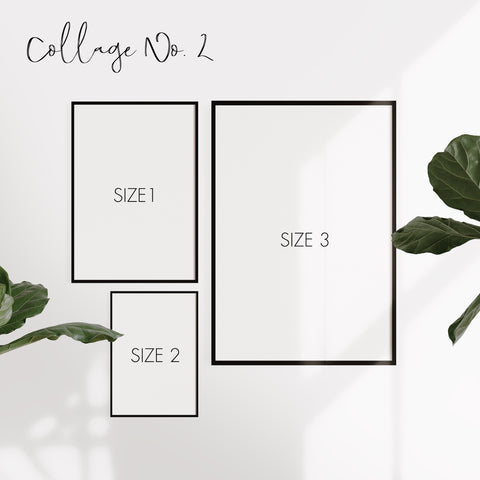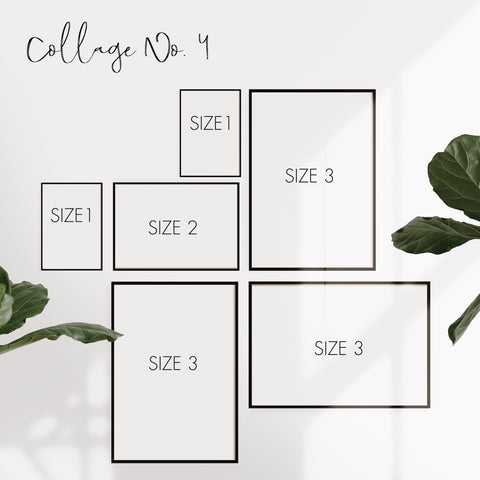# Create a wall collage

Posted by SOLT UN PEPER am

Give your walls a very special look by choosing and putting together your very own prints.

Wall collages are one way to put your favorite prints in scene afterwards.

In the following we have put together some example collages as a template or stencil.Small picture wall: Size 1 = A4 / Size 2 = A5

Middle picture wall: Size 1 = A3 / Size 2 = A4

Large picture wall: Size 1 = 50x70cm / Size 2 = 40x50cmSmall picture wall: Size 1 = A4 / Size 2 = A5 / Size 3 = A3

Middle picture wall: Size 1 = A3 / Size 2 = A4 / Size 3 = 40x50cm

Large picture wall: Size 1 = 40x50cm / Size 2 = A3 / Size 3 = 50x70cm

Large picture wall: Size 1 = 50x70cm / Size 2 = 40x50cm / Size 3 = A3Small picture wall: Size 1 = A5 / Size 2 = 40x50cm / Size 3 = A3 / Size 4 = A4

Middle picture wall: Size 1 = A4 / Size 2 = 50x70cm / Size 3 = 40x50cm / Size 4 = A3

Large picture wall: Size 1 = A3 / Size 2 = 61x91cm / Size 3 = 50x70cm / Size 4 = 40x50cmSmall picture wall: Size 1 = A5 / Size 2 = A4 / Size 3 = A3

Middle picture wall: Size 1 = A4 / Size 2 = A3 / Size 3 = 40x50cm

Large picture wall: Size 1 = A3 / Size 2 = 40x50cm / Size 3 = 50x70cm

Very large picture wall: Size 1 = 40x50cm / Size 2 = 50x70cm / Size 3 = 61x91cm